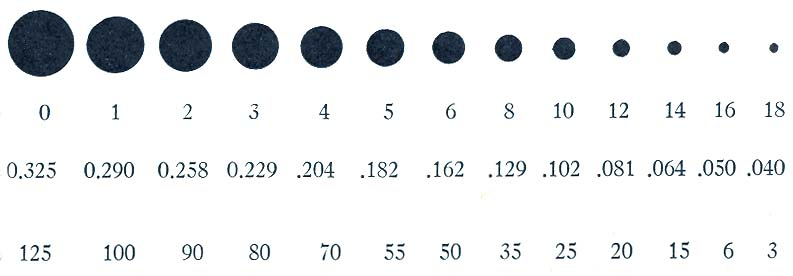# TH 1501 Stage Lighting I Electrical Theory

Parts of the Atom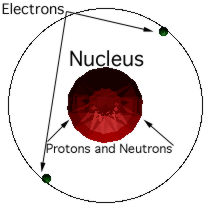Law of Changes
Protons and electrons attract.
Velocity of electrons keeps electrons in orbit.

Conductors

• Substances which have electrons that move easily from one atom to the next are considered conductors.
• Insulators are materials that resist the release of their electrons.
• Electricity always follows the path of least resistance.
• Given enough pressure (electromotive force or EMF), electricity must go somewhere.

Electrical Conductors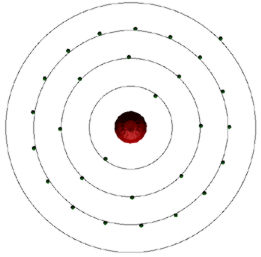Copper

Other Electrical Conductors
Silver, Brass, Aluminum

Insulators
Glass, Rubber, Fiber

Electrical Systems
• Voltage: Source of electrical potential - electromotive force.
• Current: Unbroken path (circuit) that channels flow of electrons.
• Resistance: Work or load needed to control current flow.

Ohm's Law: I = E/R

• As electromotive force increases, current increases.
As resistance increases, current decreases.

I = quantity of electrons moving through circuit measured in amperes.
E = electrical pressure in the circuit measured in voltage.
R = resistance in the circuit measured in ohms.

P = amount of work circuit is capable of providing measured in watts.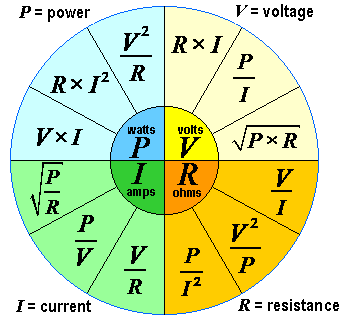West Virginia Law
W = V x A

A = current measured in amperes.
V = electromotive force measured in voltage.
W = energy available measured in wattage.

Types of Circuits
Electrical Circuits Explained

Series

Increasing amounts of resistance to same circuit, current decreases.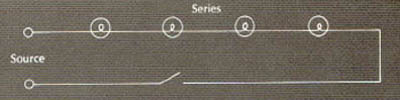Parallel
Increasing amounts of pressure to same circuit, current increases.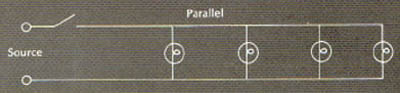Combination Circuits
Some components in series, others in parallel.

Types of Electromotive Force

Direct Current (DC)

Battery University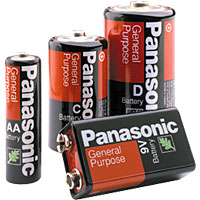Carbon Zinc Batteries General Purpose Clocks Flashlights Calculators Portable Electronics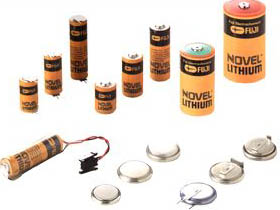Lithium Batteries Light weight High energy Portable computers Cell phones Watches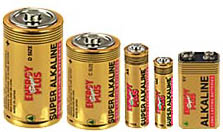Alkaline Batteries Excellent storage life Portable audio systems Cameras Toys High power flashlights Pagers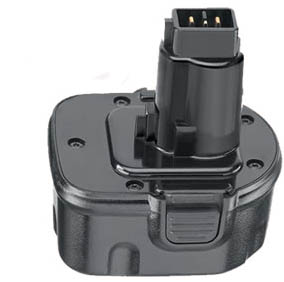Nickel Cadmium (Ni-Cad) Battery Rechargeable Recyclable Built-in charge level indicator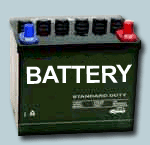Lead Acid Battery Wet cell and dry cell Cars and trucksPhotovolactic

How Alternating Current (AC) Works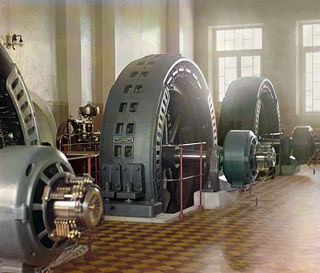Electrical Turbine

Construction of a Sine Wave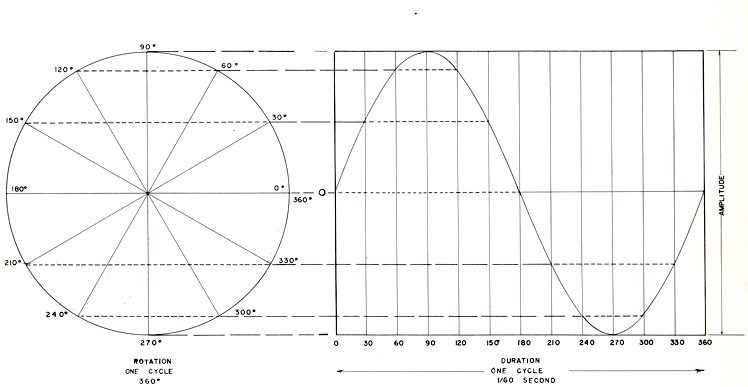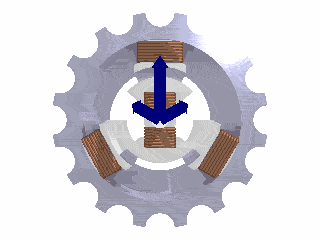Turbine Cross Section Demonstrating Rotating Magnetic Fields

United States Alternating Current Standard
Three Phase 60 Cycles per Second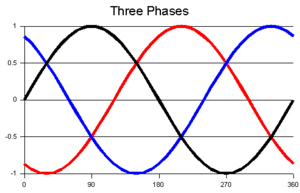Three Phase Sine Wave

Electrical Safety

Why a ground wire?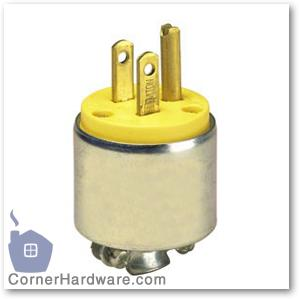Why electrical breakers?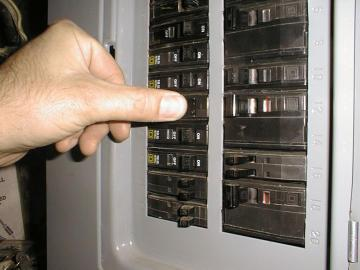Types of Wire Conductors
Solid

Stranded wire

American Wire Gauges
16 Gauge Wire: 6 amps x 120 volts = 720 watts
14 Gauge Wire: 15 amps x 120 volts = 1,680 watts

12 Gauge Wire: 20 amps x 120 volts = 2,400 watts